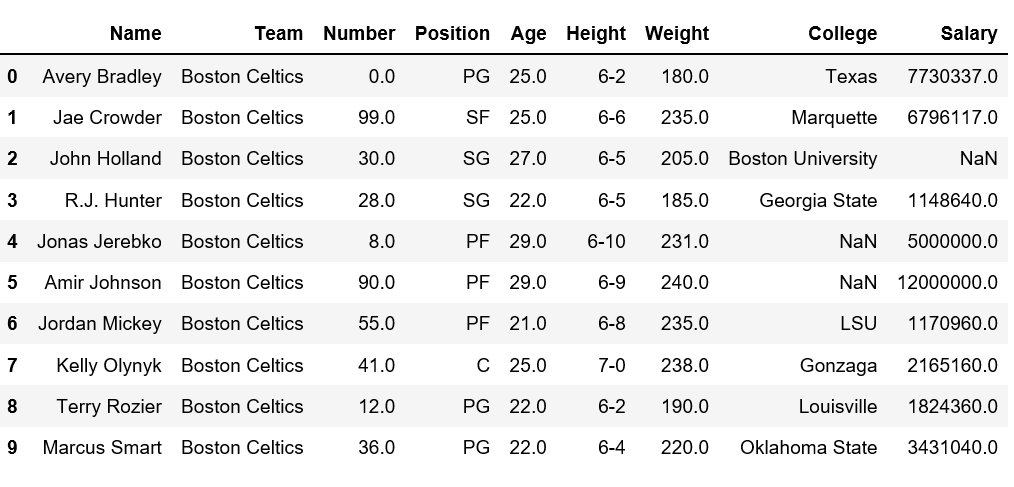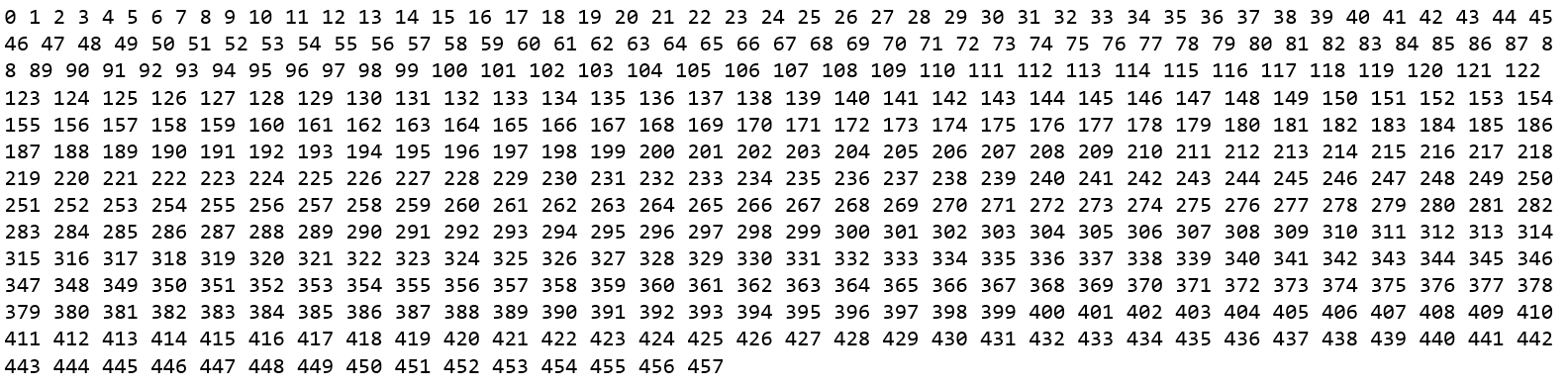Open In App

# How to get rows/index names in Pandas dataframe

While analyzing the real datasets which are often very huge in size, we might need to get the rows or index names in order to perform some certain operations.

Let’s discuss how to get row names in Pandas dataframe.

First, let’s create a simple dataframe with `nba.csv`

## Python3

 `# Import pandas package ``import` `pandas as pd ``   ` `# making data frame ``data ``=` `pd.read_csv(``"https://media.geeksforgeeks.org/wp-content/uploads/nba.csv"``) ``   ` `# calling head() method  ``# storing in new variable ``data_top ``=` `data.head(``10``) ``   ` `# display ``data_top `Now let’s try to get the row name from above dataset.

Method #1: Simply iterate over indices

## Python3

 `# Import pandas package ``import` `pandas as pd ``   ` `# making data frame ``data ``=` `pd.read_csv(``"nba.csv"``) `` ` `# calling head() method  ``# storing in new variable ``data_top ``=` `data.head() ``   ` `# iterating the columns``for` `row ``in` `data_top.index:``    ``print``(row, end ``=` `" "``)`

Output:

`0 1 2 3 4 5 6 7 8 9 `

Method #2: Using rows with dataframe object

## Python3

 `# Import pandas package ``import` `pandas as pd ``   ` `# making data frame ``data ``=` `pd.read_csv(``"nba.csv"``) `` ` `# calling head() method  ``# storing in new variable ``data_top ``=` `data.head() ``   ` `# list(data_top) or``list``(data_top.index)`

Output:

`[0, 1, 2, 3, 4, 5, 6, 7, 8, 9]`

Method #3: `index.values` method returns an array of index.

## Python3

 `# Import pandas package ``import` `pandas as pd ``   ` `# making data frame ``data ``=` `pd.read_csv(``"nba.csv"``) `` ` `# calling head() method  ``# storing in new variable ``data_top ``=` `data.head() ``   ` `list``(data_top.index.values)`

Output:

`[0, 1, 2, 3, 4, 5, 6, 7, 8, 9]`

Method #4: Using `tolist()` method with values with given the list of index.

## Python3

 `# Import pandas package ``import` `pandas as pd ``   ` `# making data frame ``data ``=` `pd.read_csv(``"nba.csv"``) `` ` `# calling head() method  ``# storing in new variable ``data_top ``=` `data.head() ``   ` `list``(data_top.index.values.tolist())`

Output:

`[0, 1, 2, 3, 4, 5, 6, 7, 8, 9]`

Method #5: Count number of rows in dataframe

Since we have loaded only 10 top rows of dataframe using `head()` method, let’s verify total number of rows first.

## Python3

 `# iterate the indices and print each one``for` `row ``in` `data.index:``    ``print``(row, end``=` `" "``)`

Output:Now, let’s print the total count of index.

## Python3

 `# Import pandas package ``import` `pandas as pd ``   ` `# making data frame ``data ``=` `pd.read_csv(``"nba.csv"``) `` ` `row_count ``=` `0`` ` `# iterating over indices``for` `col ``in` `data.index:``    ``row_count ``+``=` `1`` ` `# print the row count``print``(row_count)`

Output:

`458`